Next: Fermi surfaces and kinetic Up: Infinite Nuclear Matter Previous: Introduction

## Degrees of freedom

The four basic degrees of freedom of homogeneous INM are the isoscalar scalar density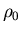, the isovector scalar density
, the isoscalar vector density s0, and the isovector vector density s1. They can be expressed through the usual neutron and proton, spin-up, and spin-down densities in the following way.=
=
s0 =
s1 =
(35)

Similarly, densities of protons and neutrons with spin up and down can be expressed as:
=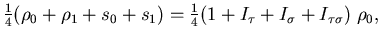=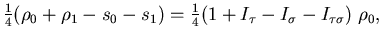=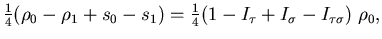=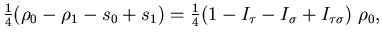where
is the relative isospin excess,
is the relative spin excess, and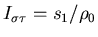is the relative spin-isospin excess, with
.

In symmetric unpolarized INM Ii = 0, while in asymmetric INM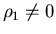. Polarized INM has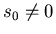, and spin-isospin polarized nuclear matter has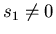.Next: Fermi surfaces and kinetic Up: Infinite Nuclear Matter Previous: Introduction
Jacek Dobaczewski
2002-03-15